Filters
Latest Questions
Physics
Pulley and string
Two masses of $4kg$ and $5kg$ are connected by a string passing through a frictionless pulley and are kept on a frictionless table as shown in figure .The acceleration of $5kg$ mass is.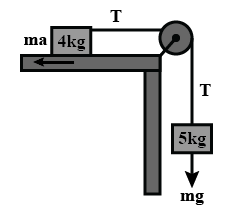Physics
Pulley and string
Pulleys and strings are massless. The horizontal surface is smooth. The acceleration of the block A is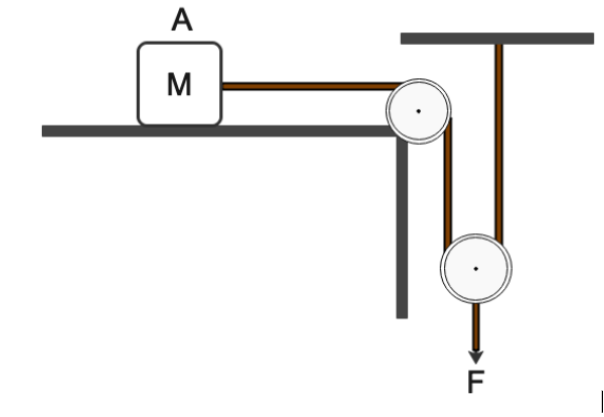$A){\text{ }}\dfrac{F}{{2M}}$
$B){\text{ }}\dfrac{F}{M}$
$C){\text{ }}\dfrac{{2F}}{M}$
$D){\text{ }}\dfrac{M}{{2F}}$
Physics
Pulley and string
A string of negligible thickness is wrapped several times around a cylinder kept on a rough horizontal surface. A man standing at a distance L from the cylinder holds one end of the string and pulls the cylinder towards him (shown in the figure). There is no slipping anywhere. The length of the string passed through the hand of the man while the cylinder reaches his hand is: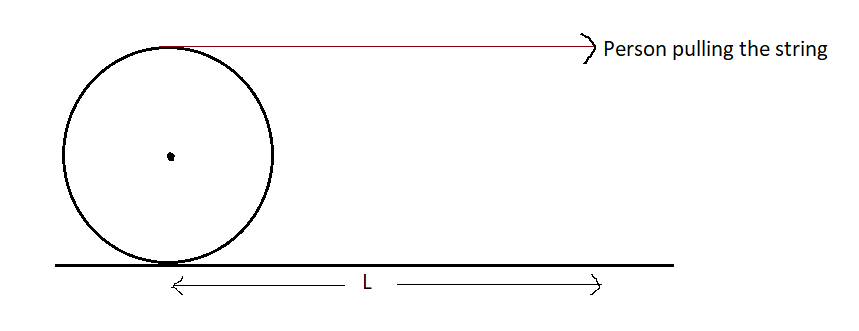A. $L$
B. $2L$
C. $3L$
D. $4L$
Physics
Pulley and string
Block B is moving towards the right with constant velocity ${v_0}$​. The velocity of block A with respect to block B is (assume all pulleys and strings are ideal):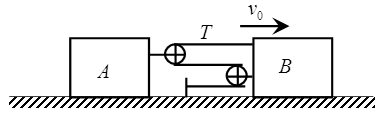A. $\dfrac{{{v_0}}}{2}$​​, left
B. $\dfrac{{{v_0}}}{2}$​​, right
C. $\dfrac{3}{{2{v_0}}}$​, right
D. $\dfrac{3}{{2{v_0}}}$​, left
Physics
Pulley and string
How do you calculate the effort force of the pulley?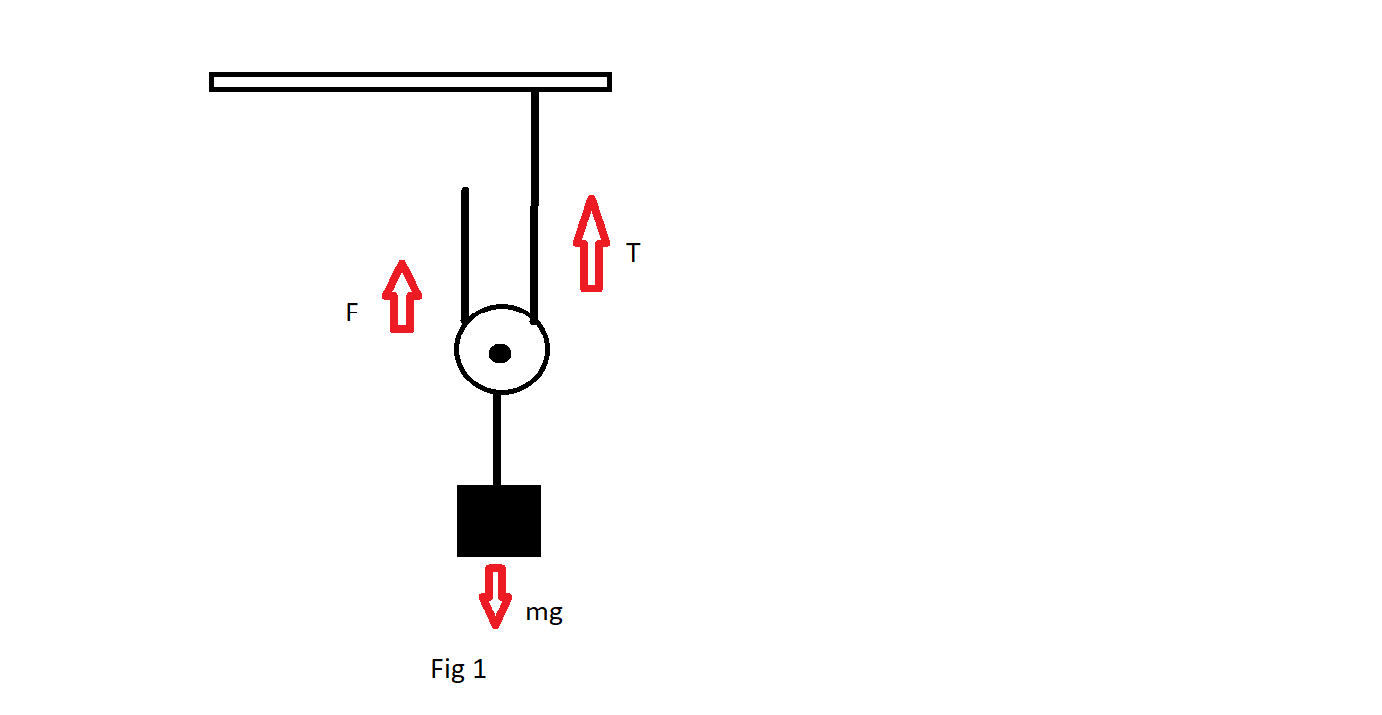Physics
Pulley and string
What does pulley mean and explain its working.
Physics
Pulley and string
How long will it take for the heavier mass to reach the floor? What will be the speed of the two masses when the heavier mass hits the floor?
An Atwood’s machine has masses of $100g$ and $110g$ . The lighter mass is on the floor and the heavier mass is $75cm$ above the floor.
Physics
Pulley and string
A light and inextensible string of length $L = 20 m$ tired between two nails, supports a frictionless pulley of weight $W_{p} = 10 \sqrt{2} N$ from which a block of weight $W_{b} = 10 \sqrt{2} N$ is also suspended as shown in the figure. The nails are fixed in a level a distance $x = 10 \sqrt{2} m$ apart. Radius of the pulley is $r = 10 cm$. How much normal reaction per unit of its length does the string apply on the pulley.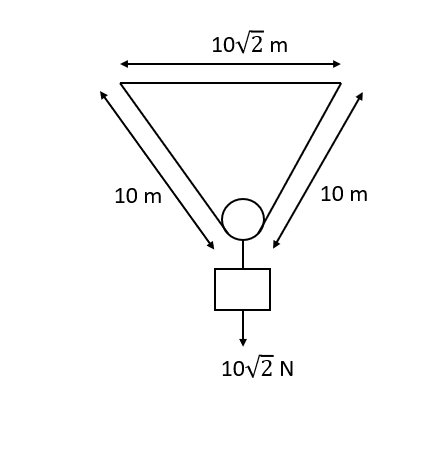Physics
Pulley and string
What is the acceleration of the block and trolley system shown in fig. if the coefficient of kinetic friction between the trolley and the surface is $0.04$? What is the tension in the string? (take $g=10\dfrac{m}{{{s}^{2}}}$). Neglect the mass of the string.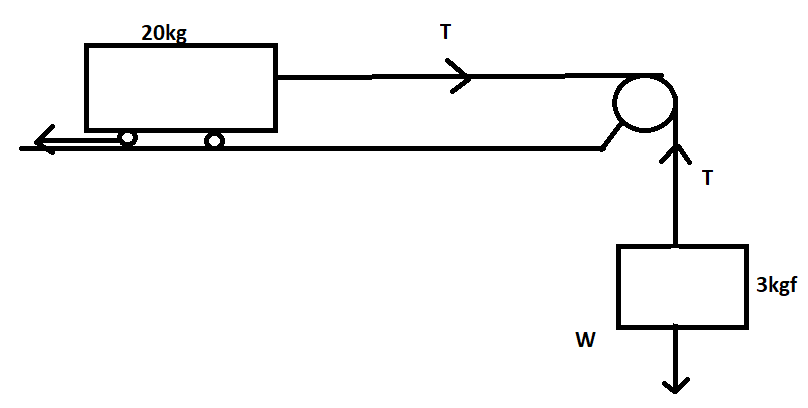Physics
Pulley and string
In the given figure a string of linear mass density m and length L is stretched by a force $F=(Fo-kt)N$ where $Fo$ & k are constant and t is time t = 0, a pulse is generated at the end P of the string. As the pulse reaches the point Q, the force vanishes. The value of K in the above equation is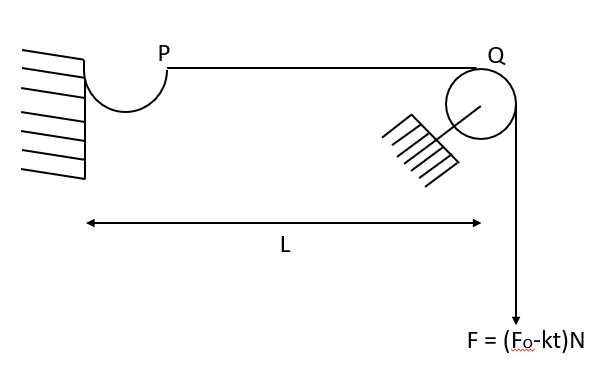Physics
Pulley and string
For the arrangement shown in the figure when the system is released, find the acceleration of the wedge. Pulley and string are ideal and friction is absent.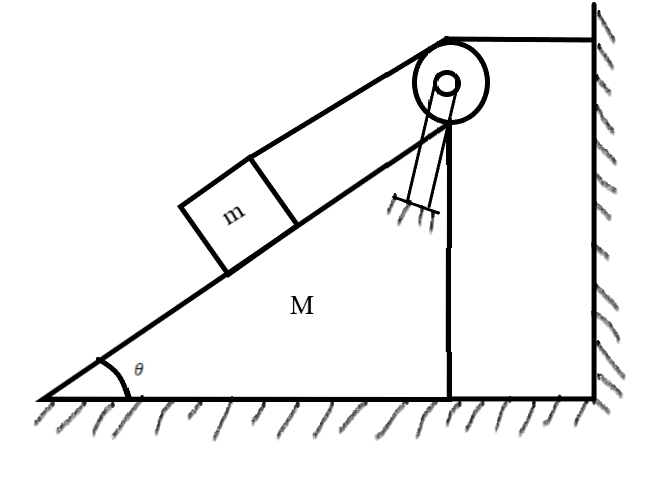Physics
Pulley and string
In the pulley system shown, if radii of the double pulley system are $2m$ and $1m$ respectively are as shown. The system is in equilibrium. The mass of two blocks A and B is :
(A) $1:5$
(B) $1:3$
(C) $1:4$
(D) $1:2$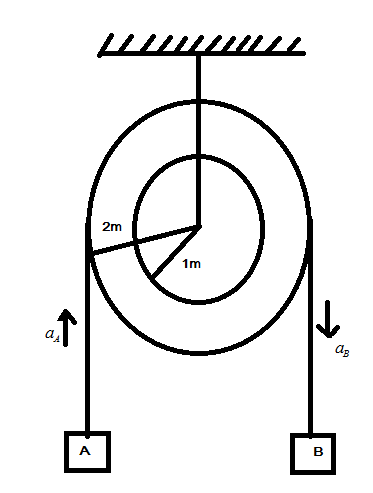Prev
1
2
3
4
5